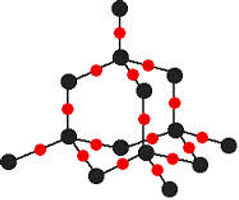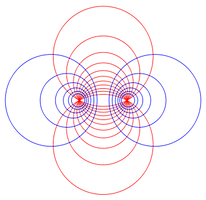## GeometryClick for...

TEACHER PLANNER

EngageNY MATH MODULE

###### Congruence, Proof, and Constructions

45-ish days

G-CO.1, G-CO.2, G-CO.3, G-CO.4, G-CO.5, G-CO.6, G-CO.7, G-CO.8, G-CO.9, G-CO.10, G-CO.11, G.CO.12, G-CO.13

In previous grades, students were asked to draw triangles based on given measurements. They also have prior experience with rigid motions—translations, reflections, and rotations—and have strategically applied a rigid motion to informally show that two triangles are congruent. In this module, students establish triangle congruence criteria, based on analyses of rigid motions and formal constructions. They build upon this familiar foundation of triangle congruence to develop formal proof techniques. Students make conjectures and construct viable arguments to prove theorems— using a variety of formats—and solve problems about triangles, quadrilaterals, and other polygons. They construct figures by manipulating appropriate geometric tools (compass, ruler, protractor, etc.) and justify why their written instructions produce the desired figure.

Click for...

TEACHER PLANNER under construction

EngageNY MATH MODULE

###### Similarity, Proof, and Trigonometry

44-ish days

G-SRT.1, G-SRT.2, G-SRT.3, G-SRT.4, G-SRT.5, G-SRT.6, G-SRT.7, G-SRT.8, G-MG.1, G-MG.2, G-MG.3

Students apply their earlier experience with dilations and proportional reasoning to build a formal understanding of similarity. They identify criteria for similarity of triangles, make sense of and persevere in solving similarity problems, and apply similarity to right triangles to prove the Pythagorean Theorem. Students attend to precision in showing that trigonometric ratios are well defined, and apply trigonometric ratios to find missing measures of general (not necessarily right) triangles. Students model and make sense out of indirect measurement problems and geometry problems that involve ratios or rates.

Click for...

TEACHER PLANNER

MODULE RESOURCES
PINTEREST BOARD

EngageNY MATH MODULE

###### Extending to Three Dimensions

10-ish days

G-GMD.1, G-GMD.3, G-GMD.4, G-MG.1,

Students’ experience with two-dimensional and three-dimensional objects is extended to include informal explanations of circumference, area and volume formulas. Additionally, students apply their knowledge of two-dimensional shapes to consider the shapes of cross-sections and the result of rotating a two-dimensional object about a line. They reason abstractly and quantitatively to model problems using volume formulas.Click for...

TEACHER PLANNER under construction

MODULE RESOURCES
PINTEREST BOARD

EngageNY MATH MODULE

###### Connecting Algebra and Geometry through Coordinates

25-ish days

G-GPE.4, G-GPE.5, G-GPE.6, G-GPE.7

Building on their work with the Pythagorean Theorem in 8th grade to find distances, students analyze geometric relationships in the context of a rectangular coordinate system, including properties of special triangles and quadrilaterals and slopes of parallel and perpendicular lines, relating back to work done in the first module. Students attend to precision as they connect the geometric and algebraic definitions of parabola. They solve design problems by representing figures in the coordinate plane, and in doing so, they leverage their knowledge from synthetic geometry by combining it with the solving power of algebra inherent in analytic geometry.Click for...

TEACHER PLANNER

MODULE RESOURCES
PINTEREST BOARD

EngageNY MATH MODULE

###### Circles with and without Coordinates

25-ish days

G-C.1, G-C.2, G-C.3, G-C.5, G-GPE.1, G-GPE.4, G-MG.1

In this module, students prove and apply basic theorems about circles, such as: a tangent line is perpendicular to a radius theorem, the inscribed angle theorem, and theorems about chords, secants, and tangents dealing with segment lengths and angle measures. They study relationships among segments on chords, secants, and tangents as an application of similarity. In the Cartesian coordinate system, students explain the correspondence between the definition of a circle and the equation of a circle written in terms of the distance formula, its radius, and coordinates of its center. Given an equation of a circle, they draw the graph in the coordinate plane and apply techniques for solving quadratic equations. Students visualize, with the aid of appropriate software tools, changes to a three-dimensional model by exploring the consequences of varying parameters in the model.